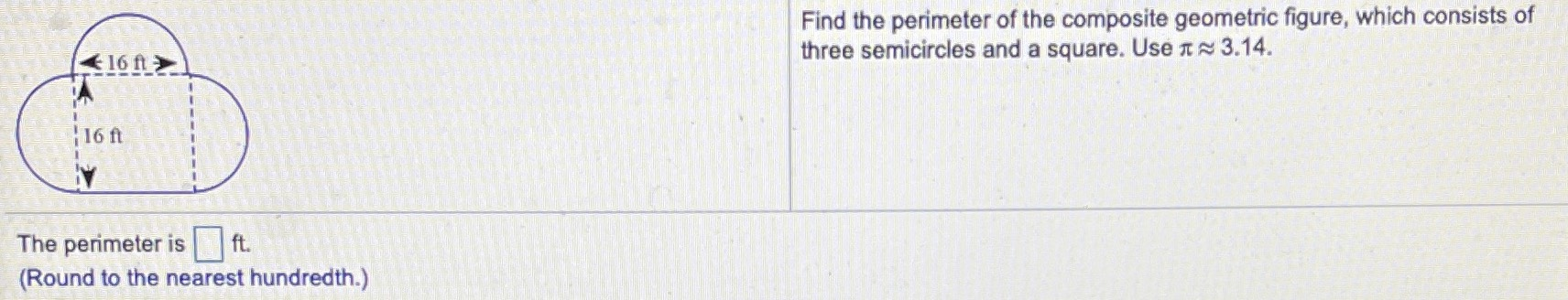Still have math questions?

Geometry
QuestionFind the perimeter of the composite geometric figure, which consists of three semicircles and a square. Use $$\pi\approx3.14$$. (Round to the nearest hundredth.)

$$91.36\text{ ft}^2$$